## Section55.6Hydrogen Atom According to Schrödinger Equation

Despite the successes of the Bohr model for Hydrogen atom it lacked any basis for the rules and important questions remained unanswered: Why would an electron not radiate when it is in these special orbits? Why can't angular momentum be any real value? Why does it have to be an integral multiple of $h/2\pi\text{?}$ Other nagging aspect of the Bohr model was that it worked well for Hydrogen atom but didn't work well for other atoms. Clearly, Bohr had something missing in his model.

Quantum mechanics provides a more satisfying treatment of the energetics of hydrogen atom. A hydrogen atom consists of a proton and an electron which interact through Coulomb force. The proton is about 2000 times more massive than the electron. We fix the proton at the origin and study the motion of the electron by quantum mechanics.

The time-independent Schrödinger equation for the wavefunction $\psi(r, \theta, \phi)$ of electron is best written in the spherical coordinates ($r,\theta,\phi$) shown in Figure 55.6.1.

\begin{equation} -\frac{\hbar^2}{2m}\nabla^2\psi - \frac{1}{4\pi\epsilon_0}\frac{e^2}{r}\psi = E \psi. \label{eq-hydrogen-atom-sch-eqn}\tag{55.6.1} \end{equation}

The solution of this equation tells us possible energy states of the electron in the hydrogen atom. It turns out that these states correspond to the Bohr orbits.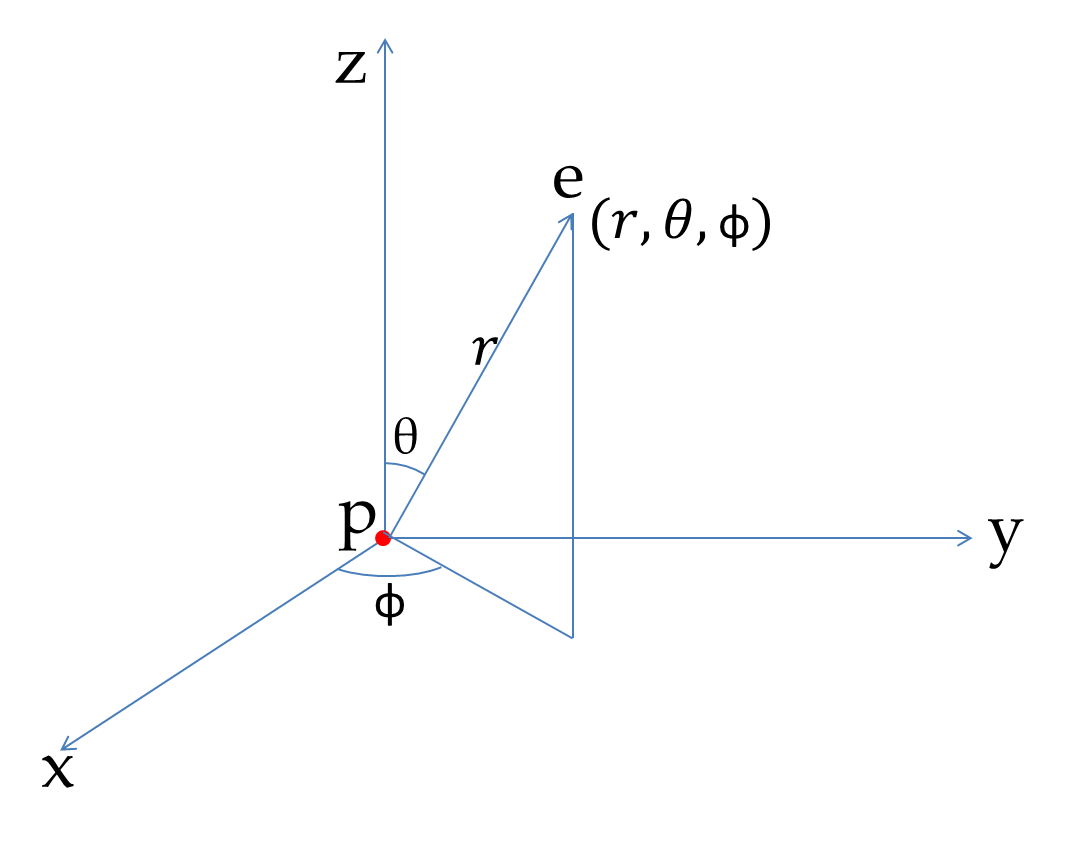Figure 55.6.1. The spherical coordinates used for the description of the wavefunction of the electron of the hydrogen atom. The proton is fixed at the origin and the coordinates of the electron is given using $(r, \theta, \phi)\text{.}$

### Subsection55.6.1Quantum Numbers of Energy States of H Atom

The solution of Eq. (55.6.1) with the condition $\psi\rightarrow 0$ as $r\rightarrow \infty$ corresponds to the states of definite energy. Although, the mathematics of working out the solution is beyond the scope of this book, we can understand the solution themselves without much mathematical complications. It turns out that there are infinitely many solutions. These solutions are labelled by three integers, $n, l, m_l\text{,}$ called quantum numbers.

\begin{equation} \psi_{n,l,m_l}(r, \theta, \phi) = R_{n,l}(r) Y_{l,m_l}(\theta, \phi),\tag{55.6.2} \end{equation}

where $R_{n,l}(r)$ is the radial part of the wavefunction and $Y_{l,m_l}(\theta,\phi)$ is the angular part of the wavefunction. In a future course you will learn more about these special functions. The functions $R_{n,l}(r)$ and $Y_{l,m_l}(\theta,\phi)$ for small values of the quantum numbers are listed in Table 55.6.2 and Table 55.6.3 respectively.

The label $n$ is called the principal quantum number, $l$ the orbital quantum number, and $m_l$ the magnetic quantum number. The principal quantum number $n$ can take on any positive integer. For a particular $n\text{,}$ the allowed values of the orbital quantum number $l$ is from $0$ to $n-1\text{.}$ For a particular $l\text{,}$ the magnetic quantum number $m_l$ can take values from $-l$ to $+l$ in steps of 1.

\begin{align*} \amp n = 1, 2, 3, \cdots\\ \amp l = 0, 1, 2, \cdots, n-1\\ \amp m_l = -l, -(l-1), -(l-2), \cdots, l-2, l-1, l. \end{align*}

The energy $E_{n,l,m_l}$ of the state $\psi_{n,l,m_l}$ with quantum numbers $n, l, m_l$ depends only on the principal quantum number $n\text{.}$

\begin{equation} E_{n,l,m_l} = -\frac{1}{4\pi\epsilon_0}\: \frac{e^2}{a_0}\:\frac{1}{n^2} \equiv -\frac{E_{1,0,0}}{n^2}. \label{eq-hydrogen-energy}\tag{55.6.3} \end{equation}

where $a_0$ is the Bohr radius defined in Eq. (55.5.6). Note that this is the values of energy of the stationary states obtained by Bohr as given in Eq. (55.5.10). Since the energy of the hydrogen atom does not depend on $l$ or $m_l$ we often write $E_n$ for $E_{n,l,m_l}\text{.}$

\begin{equation*} E_n \equiv E_{n,l,m_l}. \end{equation*}

All states with the same $n$ value are said to form a shell and various states within this group with same $l$ are said to form a subshell. Historically, shells and subshells were designated by spectroscopic notation with capital letters for shells and small letters for subshells. Thus, $n=1,\ 2,\ 3,\ 4,\ 5,\ 6, \cdots$ are designated by $K,\ L,\ M,\ N,\ O,\ P,\ \cdots$ and $l = 0,\ 1,\ 2,\ 3,\ 4,\ 5, \cdots$ are designated by $s,\ p,\ d,\ f,\ g,\ \cdots$ as illustrated in Figure 55.6.4.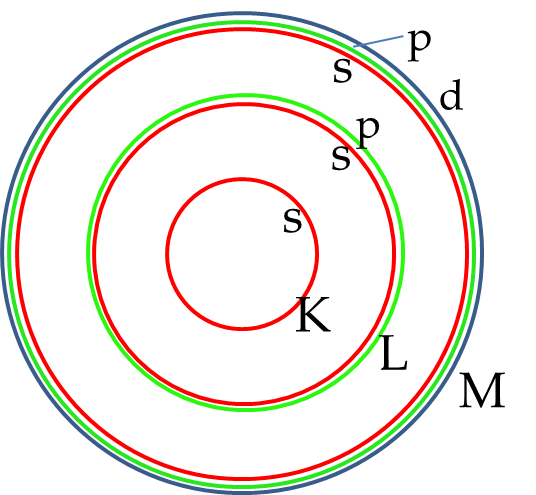Figure 55.6.4. The shells and subshells for electronic states in hydrogen atom. The $n=1,\ 2,\ 3, \cdots$ are called shells $K,\ L,\ M, \cdots\text{,}$ and the $l=0,\ 1,\ 2, \cdots$ are called subshells $s,\ p,\ d, \cdots\text{.}$

An energy level diagram helps visualize the energies of states. Figure 55.6.5 shows the energy diagram for the hydrogen atom corresponding the energies in Eq. (55.6.3). In the energy level diagram, we use a mixed notation: use $n$ for the shell and spectroscopic notation $s,\ p,\ d,\ f,\ g,\ \cdots$ for the subshells.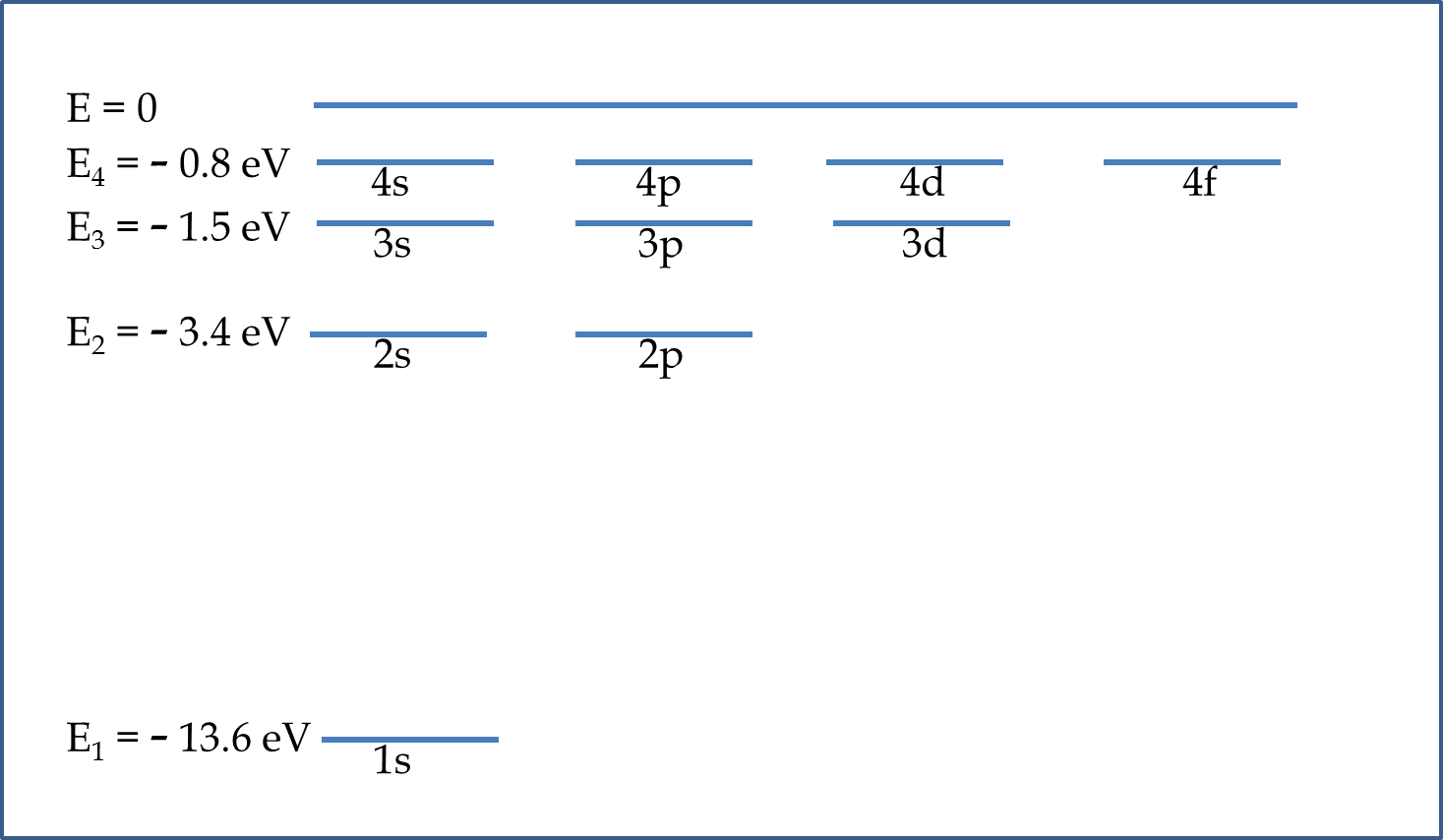Figure 55.6.5. The energy level diagram for the hydrogen atom. Note that reference is chosen so that when energy is greater than 0, the electron will not be bound to the proton.

### Subsection55.6.2Low-Energy States of Hydrogen Atom

The Ground State

The lowest energy state is called the ground state. According to Eq. (55.6.3), the lowest energy of hydrogen atom corresponds to $n=1\text{.}$ The other quantum numbers for $n=1$ will be $l=0$ and $m_l = 0\text{.}$ The energy of this state is given by

\begin{equation} E_{1, 0, 0} = -\frac{1}{4\pi\epsilon_0}\: \frac{e^2}{a_0}\:\frac{1}{1^2} = - 13.6\:\textrm{eV}.\tag{55.6.4} \end{equation}

The wavefunction for the ground state $\psi_{1,0,0}(r,\theta,\phi)$ is given by the product of the radial and angular parts as stated above.

\begin{equation} \psi_{1,0,0} = R_{1,0} Y_{0,0} = \frac{1}{2\sqrt{\pi}}\: \left(\frac{1}{a_0}\right)^{3/2} 2\: e^{-r/a_0}.\tag{55.6.5} \end{equation}

Thus, the wavefunction for the ground state is isotropic in space, i.e., it is independent of the direction in space. The reason for the isotropy can be traced to the isotropy of the potential energy in the Schrödinger equation. The wave function for the ground state has the largest value at the origin, where the nucleus is located and decreases exponentially with distance.

The ground state wave function of hydrogen atom is also real. Its square gives the probability density of finding the electron in a small volume around a point. Thus, in a small volume $dV$ around the point $(r,\theta,\phi)$ the probability that the electron is in this volume will be

\begin{equation} dP = \left|\psi_{1,0,0} \right|^2 dV.\tag{55.6.6} \end{equation}

The volume element in spherical coordinates is

\begin{equation} dV = r^2 \sin\theta dr d\theta d\phi.\tag{55.6.7} \end{equation}

Since $\psi_{1,0,0}$ is only a function of $r$ we can deduce the probability of finding the electron in the spherical shell of thickness $dr$ at distance $r$ by integrating over $\theta$ and $\phi\text{.}$ Let us denote this probability as $dP_r$

\begin{equation} dP_r = \left|\psi_{1,0,0} \right|^2 4\pi r^2 dr = \frac{4}{a_0^3}\: r^2 e^{-2r/a_0} dr. \label{eq-hydrogen-probability-1s-1}\tag{55.6.8} \end{equation}

The quantity multiplying $dr$ is the probability per unit distance from the nucleus. Let us denote this quantity by symbol $\rho_{1s}$ to indicate that it is a density for the $1s$ state.

\begin{equation} \rho_{1s} (r)= \frac{4}{a_0^3}\: r^2 e^{-2r/a_0}. \label{eq-hydrogen-electron-cloud-probability}\tag{55.6.9} \end{equation}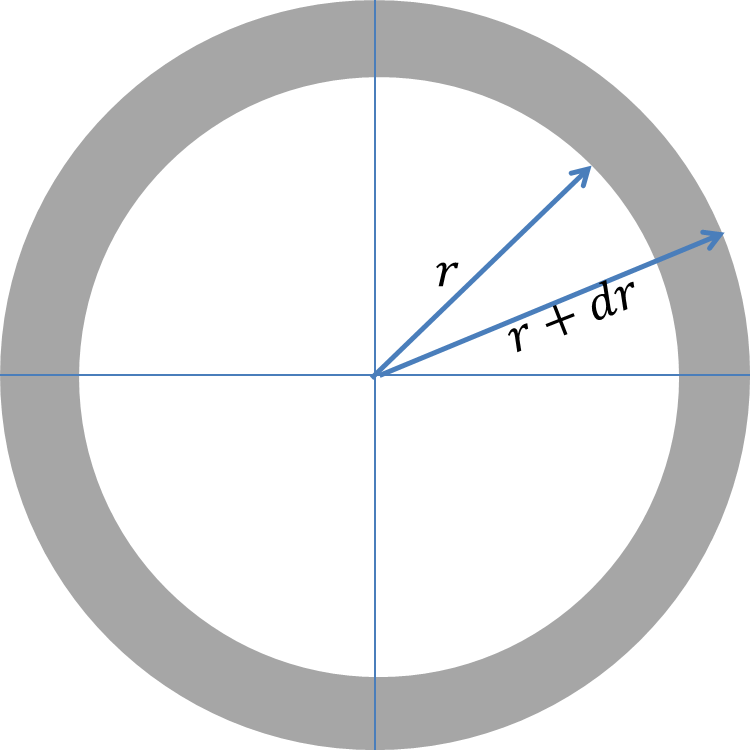Figure 55.6.6. The probability that the electron is in the shaded region is given by $dP_r =\rho_{1s}(r) dr\text{,}$ where $\rho_{1s}$ is given in Eq. (55.6.9).

Note that the probability in Eq. (55.6.8) is properly normalized. That is, the probability of finding the electron somewhere in space is equal to 1, as you can verify by integrating the right side from $r=0$ to $r=\infty\text{.}$

\begin{equation} \int_0^{\infty}\rho_{1s} (r)dr = \int_0^{\infty}\frac{4}{a_0^3}\: r^2 e^{-2r/a_0} dr = 1.\tag{55.6.10} \end{equation}

A plot of $\rho_{1s} (r)$ versus the radial distance $r$ shown in Figure 55.6.7 is instructive as it shows visually how the probability per unit distance varies with distance from the nucleus. The peak of this plot occurs at the Bohr radius, $r=a_0\text{.}$ We say that the most probable distance to find the electron from the nucleus is $r = a_0\text{.}$

Note the departure of the quantum mechanical interpretation from that of the Bohr model. While, in the Bohr model, we had a definite orbit for the electron, in quantum mechanics, the electron can be anywhere with different probabilities. For the lowest energy state, the most probable distance the electron can be found is at the Bohr radius.

Since the electron has different probabilities for being at different distances from the nucleus we can calculate the average distance of the elctron from the nucleus by averaging the variable $r$ with respect to the probability distribution $\rho_{1s} (r)\text{.}$

\begin{equation} \langle r \rangle = \int_0^{\infty}r\:\rho_{1s} dr = \frac{4}{a_0^3}\int_0^{\infty}r^3 e^{-2r/a_0} dr = \frac{3}{2} a_0.\tag{55.6.11} \end{equation}

Although the probability density peaks at $r=a_0\text{,}$ the average $r$ is at $r= \dfrac{3}{2}a_0\text{.}$Figure 55.6.7. Probability density of finding an electron at a distance $r$ from the nucleus of a hydrogen atom. [Note to publisher: A picture of $\psi^2$ for 1s state will be good here.]

First excited state

The energy of the first excited occurs when the principal quantum number has the value $n=2\text{.}$ The orbital quantum numbers for $n=2$ has two possible values, $l=0,1\text{.}$ When $l=0\text{,}$ $m_l$ has only one value, $m_l =0\text{.}$ When $l=1\text{,}$ $m_l$ has three possible values and $m_l = -1,0,1\text{.}$ Therefore, we have four different possible states for the energy state $E_2\text{.}$

\begin{align*} \amp n = 2,\ l = 0,\ m_l = 0,\\ \amp n = 2,\ l = 1,\ m_l = -1,\\ \amp n = 2,\ l = 1,\ m_l = 0,\\ \amp n = 2,\ l = 1,\ m_l = 1. \end{align*}

All four states have the same energy since they all have $n=2$ and the energy depends only on the value of $n\text{.}$

\begin{equation*} E_{2, l, m_l} = -\frac{1}{4\pi\epsilon_0}\: \frac{e^2}{a_0}\:\frac{1}{2^2} = -3.4\:\textrm{eV}. \end{equation*}

Since four states have the same energy we say that the state is degenerate with degeneracy four. The wavefunctions for the four states are different.

\begin{align*} \amp \psi_{2,0,0} = R_{2,0} Y_{0,0}, \\ \amp \psi_{2,1,-1} = R_{2,1} Y_{1,-1},\\ \amp \psi_{2,1,0} = R_{2,1} Y_{1,0},\\ \amp \psi_{2,1,1} = R_{2,1} Y_{1,1}, \end{align*}

where the $R$'s and $Y$'s are given in Table 55.6.2 and Table 55.6.3 respectively. From the Tables we find that while $\psi_{2,0,0}\text{,}$ called the $2s$ orbital is isotropic, the other states $\psi_{2,1,-1}\text{,}$ $\psi_{2,1,0}\text{,}$ $\psi_{2,1,1}\text{,}$ collectively called $2p$ states are not isotropic. Even though the wave functions for $2s$ and $2p$ states of the hydrogen atom are very different in their distribution in space, the energies corresponding to these states are equal.

What is the probability of finding the electron in the 1s state to be within a distance $a_0$ of the nucleus?

Hint

Integrate probability density.

$0.32\text{.}$

Solution

The integration of the probability density $\rho_{1s}$ from $r=0$ to $r=a_0$ will give us the required probability.

\begin{equation*} P_{r\le a_0} = \int_0^{a_0} \rho_{1s} dr = \int_0^{a_0} \frac{4}{a_0^3}\: r^2 e^{-2r/a_0} dr = 1 - \frac{5}{e^2} \approx 0.32. \end{equation*}

That is, there is only 32\% chance that the electron is within a Bohr radius if the electron is in the 1s state.

### Subsection55.6.3Emission Spectrum of Hydrogen Atom

The emission of photons are connected to the pumping of a system from one energy state to another energy state of lower energy. The absorption happens when an incident photon is able to cause the transition of a system from a lower energy state to a higher energy state. Figure 55.6.9 shows transition of states $(n=2, l=1,m_l=0,\pm1)$ to state $(n=1, l=0,m_l=0)$ and states $(n=3, l=2,m_l=0,\pm1, \pm2)$ to states $(n=1, l=1,m_l=0, \pm1)\text{.}$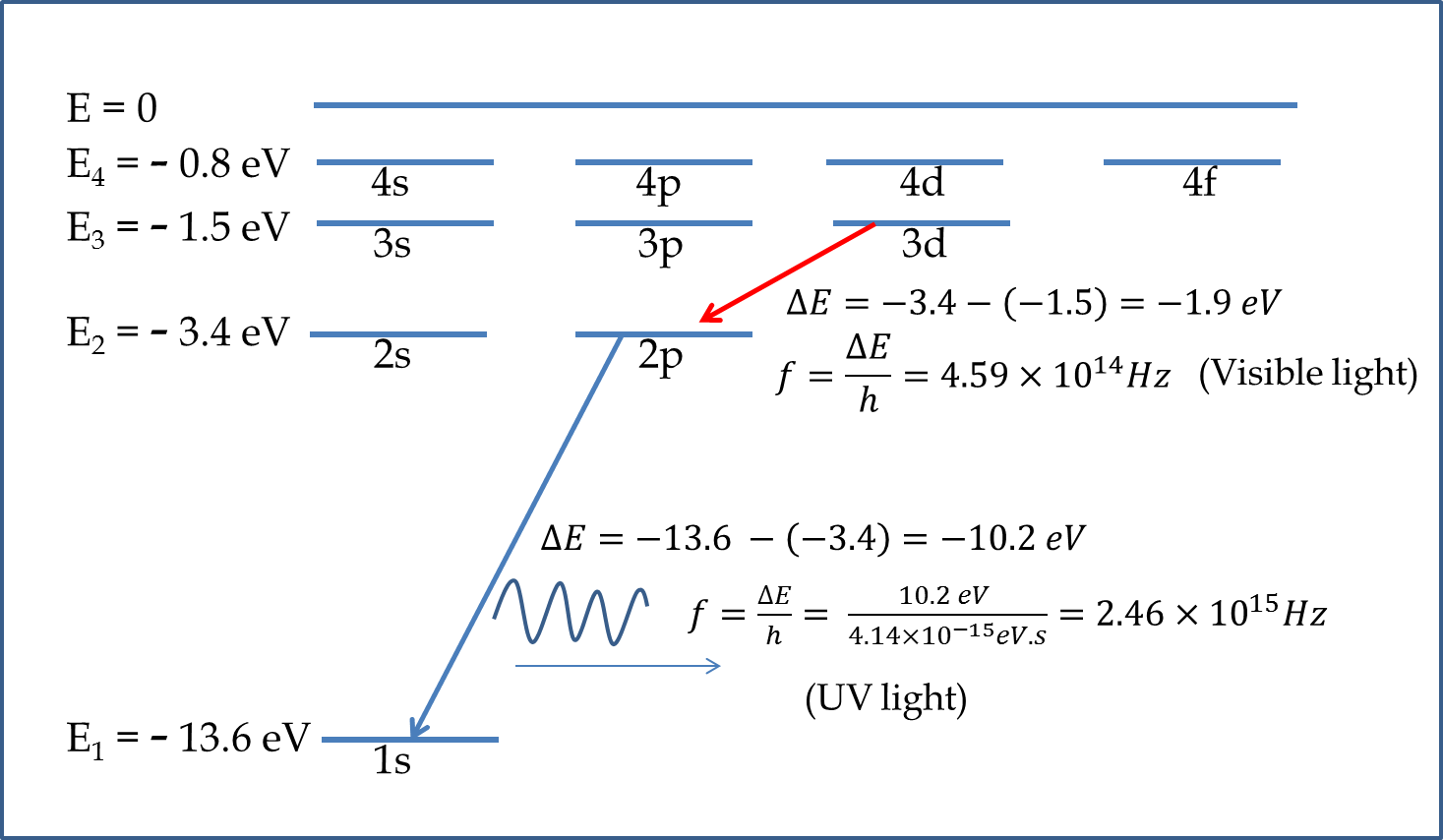Figure 55.6.9. The transition hydrogen atom between its stationary states results in emission of light. The emission for 3d state to 2p state transition emits light in the visible range while the emission from the 2p state to 1s state is in the ultraviolet range.

The energy conservation dictates that the energy of the photon released must equal the change in the energy of the electron. Therefore, if an electron jumps from a state of energy $E_n$ to a state of energy $E_m \lt E_n\text{,}$ the energy of the emitted photon will be the difference $E_n-E_m\text{.}$ Equating this to $hf$ gives us the frequency of the light emitted in the process.

\begin{equation} h f = E_n-E_m.\tag{55.6.12} \end{equation}

We can obtain the wavelength of the light in the process by writing $\lambda$ in terms of $f$ and the speed of light $c\text{.}$

\begin{equation} \lambda = \frac{c}{f} = \frac{hc}{E_n-E_m}.\tag{55.6.13} \end{equation}

Among all the transitions of hydrogen atom, the transition of the hydrogen atom to $n=2$ state from states $n=3,4,5,6$ are particularly interesting since the energy corresponds to the release of photons in the visible spectrum. The series is called the Balmer series as we have seen before.

What is the energy and wavelengths of the photons released when hydrogen atom jumps from a state with $n=4$ to $n=2\text{?}$

Hint

Solution

The energy of the photon will be the difference in the energy of the two states of hydrogen.

\begin{equation*} E_{\textrm{photon}} = -\frac{E_1}{4^2} - (-\frac{E_1}{2^2}) = \frac{3}{16} E_1 = \frac{3}{16} \times 13.6\:\textrm{eV} = 2.55\:\textrm{eV}. \end{equation*}

The wavelength of the photon will be

\begin{equation*} \lambda = \frac{hc}{E_{\textrm{photon}}} = \frac{1.24\:\textrm{eV.}\mu\textrm{m}}{2.55\:\textrm{eV}} = 0.486\ \mu\textrm{m}. \end{equation*}

### Subsection55.6.4Quantization of Energy and Angular Momentum

The solution of the hydrogen atom problem has shown us that the energy of hydrogen atom can take only certain discrete value, labeled by the principal quantum number $n\text{.}$

\begin{equation} E_n = - \frac{E_1}{n^2}, \ \ n = 1, 2, 3, \cdots.\tag{55.6.14} \end{equation}

The quantum states are labeled with two additional quantum numbers, $(l, m_l)\text{,}$ that inform us about the angular variation of the wavefunction. A more detail analysis shows that these quantum numbers give us the information about the magnitude of the angular momentum $|\vec L|$ and the component of the angular momentum about a particular direction in space, which is usually taken to be the $z$-axis, $L_z\text{.}$ A state $\psi_{n,l,m_l}$ has the following magnitude of the angular momentum and the $z$-component of the angular momentum.

\begin{align} \amp |\vec L| = \sqrt{l(l+1)}\: \hbar,\tag{55.6.15}\\ \amp L_z = m_l \hbar,\tag{55.6.16} \end{align}

where $\hbar = h/2\pi\text{.}$ Recall that for a particular $n\text{,}$ the $l$ takes integer values from $0$ to $n-1$ and for a particular $l\text{,}$ the $m_l$ takes integer values between $-l$ and $l\text{.}$ Therefore, $|\vec L|$ and $L_z$ take on discrete values. We say that $|\vec L|$ and $L_z$ are quantized.

The quantization of the $z$ (or $x\text{,}$ or $y$) component of the angular momentum is also called space quantization. Since $L_z=|\vec L|\cos\theta$ for angular momentum vector pointed at angle $\theta$ to the $z$-axis, the quantization of $L_z$ means quantization of $\theta\text{,}$ the direction in space, hence the term space quantization. Each allowed value of $L_z$ for a particular value of $|\vec L|$ corresponds to the angular momentum vector pointed in specific direction in space. That is, quantum mechanics of hydrogen atom shows that the angular momentum of the electron in the atom must be pointed only at certain angles to the chosen axis.

(a) An electron in the hydrogen atom has $l=2\text{,}$ what is the magnitude of the angular momentum? (b) What are the possible values of $m_l$ for this electron? (c) What are the possible values of the $z$-component of the angular momentum vector for this electron? (d) What directions in space can the angular momentum vectors be pointed? (e) Draw a diagram showing the directions of the angular momentum vectors in space.

Hint

Solution

(a) This is just a straighforward application of the given formula for $|\vec L|\text{.}$

\begin{equation*} |\vec L|= \sqrt{l(l+1)}\: \hbar = \sqrt{2(2+1)}\: \hbar = \sqrt{6}\: \hbar = 2.6 \times 10^{-34}\: J.s. \end{equation*}

(b) $m_l$ takes values from $-l$ to $+l\text{.}$ Therefore, the possible values of $m_l$ of this electron will be

\begin{equation*} m_l = -2,\ -1,\ 0,\ 1,\ 2. \end{equation*}

(c) This is just a straighforward application of the given formula for $L_z$ for each value of $m_l\text{.}$

\begin{equation*} L_z = m_l \: \hbar = \left( -2\hbar,\ -1\hbar,\ 0,\ 1\hbar,\ 2\hbar\right) = (-2.1,\ - 1.05,\ 0 ,\ 1.05,\ 2.1) \times 10^{-34}\: J.s. \end{equation*}

(d) We work out the angles by setting $\cos\theta = L_z/L$ with $L= \sqrt{6}\: \hbar$ and $L_z = \left( -2\hbar,\ -1\hbar,\ 0,\ 1\hbar,\ 2\hbar\right)\text{.}$ You should get

\begin{equation*} \cos\theta = \left( -\frac{2}{\sqrt{6}},\ -\frac{1}{\sqrt{6}},\ 0,\ \frac{1}{\sqrt{6}},\ \frac{2}{\sqrt{6}}\right). \end{equation*}

Therefore, the angles are

\begin{equation*} \theta = -35.3^{\circ},\ -65.9^{\circ},\ 0,\ 65.9^{\circ},\ 35.3^{\circ}. \end{equation*}

(e) The five quantized directions in space are shown by the five angular momentum arrows for $l=2\text{.}$ The rotation of the arrows about the $z$-axis give all other directions in space for the angular momentum vector.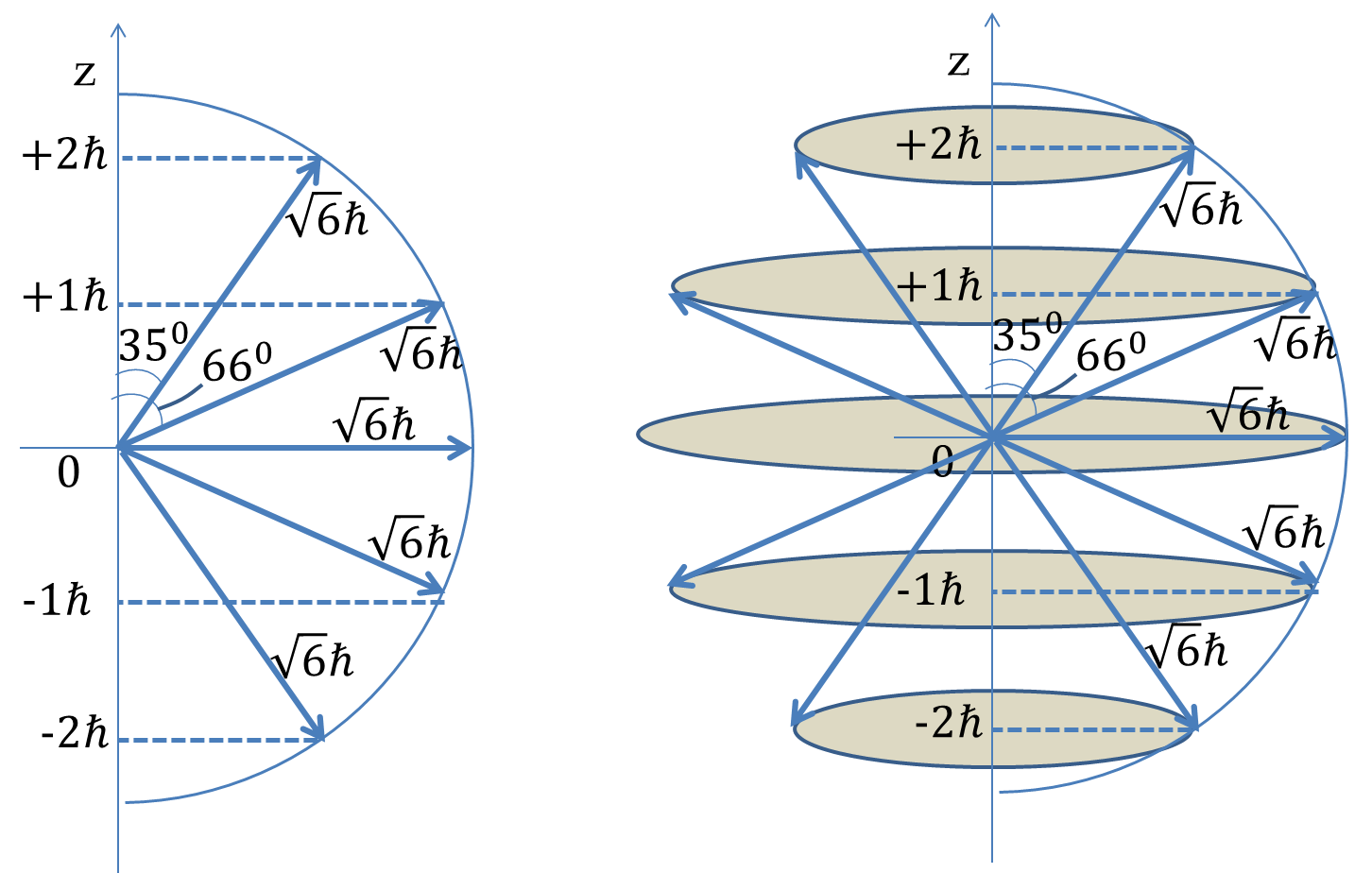The electron in a hydroen atom is in a quantum state with principal quantum number $n=4\text{.}$ (a) What are the possible values of the orbital angular momentum $l\text{?}$ (b) For each possible values of $l\text{,}$ what are the possible values of the magnetic quantum number $m_l\text{?}$ (c) How many quantum states of hydrogen atom are possible that have $n=4$ as their principal quantum number [ignoring the spin for now]?

(a) An electron is in s subshell of the $K$ shell. What are its $n$ and $l\text{?}$ (b) An electron has $n=3$ and $l=2\text{,}$ what are the spectroscopic letter designations of the shell and subshell for these values?

The electron in the hydrogen atom is in the 1s state. (a) What is the probability that the electron is located within $a_0/4$ of the nucleus? (b) What is the probability that the electron is outside a distance $r=4a_0$ from the nucleus? Here $a_0$ is the Bohr radius.

The electron in the hydrogen atom is in the state $n=2, l=1, m_l = 0\text{.}$ (a) What is the probability that the electron is located within $a_0$ of the nucleus? (b) What is the probability that the electron is outside a distance $r=2a_0$ from the nucleus? Here $a_0$ is the Bohr radius.

The electron in the hydrogen atom is in the state $n=2, l=1, m_l = 0\text{.}$ (a) What is the probability that the electron is located in the direction $\theta \le 45^{\circ}$ of the $z$-axis? (b) What is the probability that the electron is located in the direction $\theta \le 45^{\circ}$ of the $xy$-plane?

The electron in the hydrogen atom is in the state $n=2, l=1, m_l = +1\text{.}$ (a) What is the probability that the electron is located within $a_0$ of the nucleus? (b) What is the probability that the electron is outside a distance $r=2a_0$ from the nucleus? Here $a_0$ is the Bohr radius.

The electron in the hydrogen atom is in the state $n=2, l=1, m_l = +1\text{.}$ (a) What is the probability that the electron is located in the direction $\theta \le 45^{\circ}$ of the $z$-axis? (b) What is the probability that the electron is located in the direction $\theta \le 45^{\circ}$ of the $xy$-plane?

An electron makes a transition from 3p state to 2s state of a Hydrogen atom. What will be the energy and wavelength of the photon emitted?

An electron makes a transition from 6d state to 5p state of Hydrogen atom. What will be the energy and wavelength of the photon emitted?